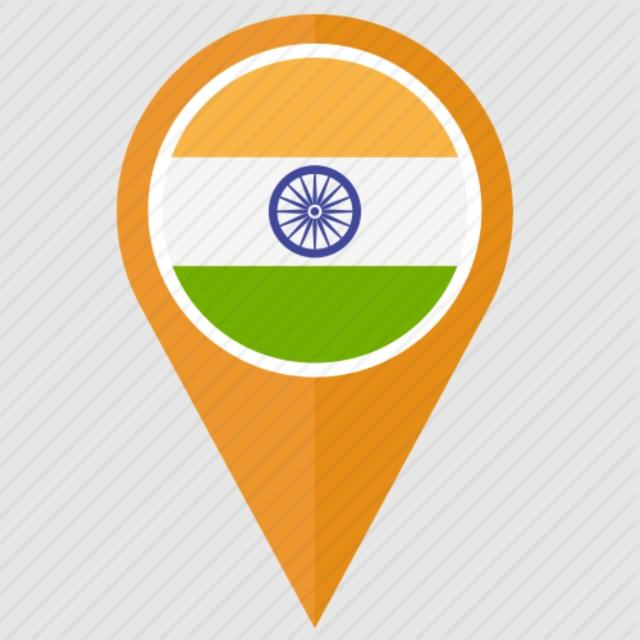# Why are all points displaying in the ocean near Africa?

10580
4
09-30-2017 05:51 PMNew Contributor II

Creating centroids from a world country polygon layer. After displaying the XY Data all points show up in the ocean near Africa? I verified all geographic and projected coordinate systems are the same. See full workflow above.

1 Solution

Accepted SolutionsbyMVP Esteemed Contributor

Rightly pointed by Dan, it's an issue with the Coordinate System - Coordinate Values mismatch.

WGS_84_Web_Mercator (Auxiliary Sphere) is a Projected Coordinate System. Thus, its units are in Meters. When you add the values (in Decimal Degrees) using Web_Mercator, it considers the units to be in Meters, instead of Decimal Degrees.

Here is what you need to do (choose any one of the following).

1. While using Calculate Geometry for calculating Centroid, you can keep the default units, i.e., don't change the units to decimal degrees. Then the values will be correct when you add data in WGS_84_Web_Mercator (Auxiliary Sphere).

2. If you want the XY values in Decimal Degrees (Calculate Geometry), then choose the Spatial Reference as GCS_WGS_1984 while Adding XY Data (as pointed by Dan).

3. If you have ArcGIS Desktop Advanced License, you could use Feature To Point—Help | ArcGIS Desktop

Think Location
4 RepliesbyMVP Legendary Contributor

The coordinates are being treated as planar values.

When you created the event layer, you should have defined its coordinate system as a Geographic Coordinate System, most likely a GCS WGS84.  Without the definition of what it is, it won't be projected to what you want it to do.  I would also suggest that you move on from an event layer and save/export it to either a geodatabase featureclass or shapefile.

As a side note, make sure that you get the centroidX and centroidY the rightway around (X is longitude and Y is latitude... not quite sure how the centroid values were created)New Contributor II

Thanks for the quick response Dan!byMVP Esteemed Contributor

Rightly pointed by Dan, it's an issue with the Coordinate System - Coordinate Values mismatch.

WGS_84_Web_Mercator (Auxiliary Sphere) is a Projected Coordinate System. Thus, its units are in Meters. When you add the values (in Decimal Degrees) using Web_Mercator, it considers the units to be in Meters, instead of Decimal Degrees.

Here is what you need to do (choose any one of the following).

1. While using Calculate Geometry for calculating Centroid, you can keep the default units, i.e., don't change the units to decimal degrees. Then the values will be correct when you add data in WGS_84_Web_Mercator (Auxiliary Sphere).

2. If you want the XY values in Decimal Degrees (Calculate Geometry), then choose the Spatial Reference as GCS_WGS_1984 while Adding XY Data (as pointed by Dan).

3. If you have ArcGIS Desktop Advanced License, you could use Feature To Point—Help | ArcGIS Desktop

Think LocationNew Contributor II

Thank you for the detailed response! I am a novice user so providing the steps I needed to fix the issue was awesome!